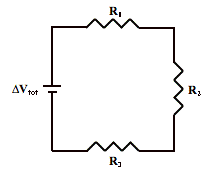# Series circuit problemThe series circuit at the right depicts three resistors connected to a voltage source. The voltage source (ΔVtot) is a 110-V source and the resistor values are 7.2 Ω (R1), 6.2 Ω (R2) and 8.6 Ω (R3). -Determine the equivalent resistance of the circuit.

×